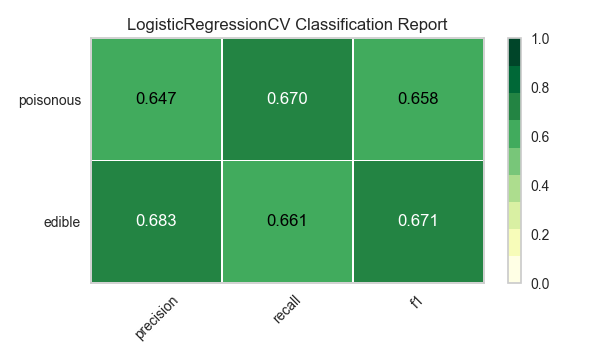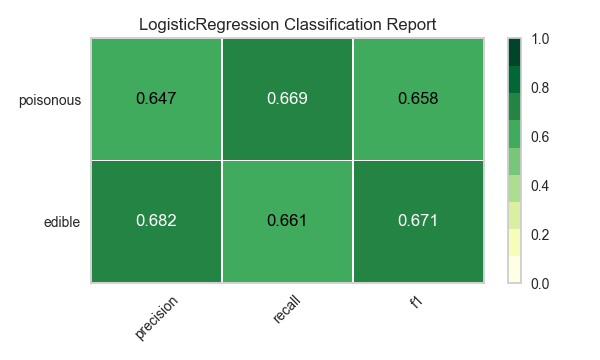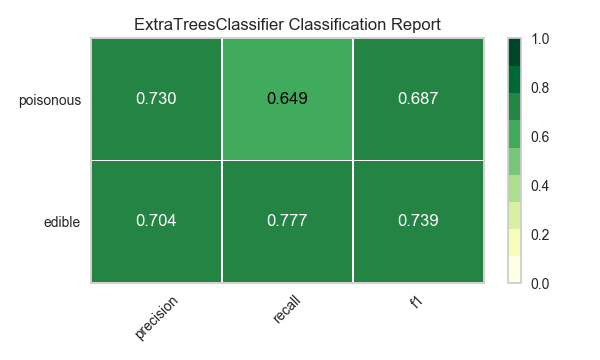# Model Selection Tutorial¶

In this tutorial, we are going to look at scores for a variety of Scikit-Learn models and compare them using visual diagnostic tools from Yellowbrick in order to select the best model for our data.

## The Model Selection Triple¶

Discussions of machine learning are frequently characterized by a singular focus on model selection. Be it logistic regression, random forests, Bayesian methods, or artificial neural networks, machine learning practitioners are often quick to express their preference. The reason for this is mostly historical. Though modern third-party machine learning libraries have made the deployment of multiple models appear nearly trivial, traditionally the application and tuning of even one of these algorithms required many years of study. As a result, machine learning practitioners tended to have strong preferences for particular (and likely more familiar) models over others.

However, model selection is a bit more nuanced than simply picking the “right” or “wrong” algorithm. In practice, the workflow includes:

1. selecting and/or engineering the smallest and most predictive feature set
2. choosing a set of algorithms from a model family, and
3. tuning the algorithm hyperparameters to optimize performance.

The model selection triple was first described in a 2015 SIGMOD paper by Kumar et al. In their paper, which concerns the development of next-generation database systems built to anticipate predictive modeling, the authors cogently express that such systems are badly needed due to the highly experimental nature of machine learning in practice. “Model selection,” they explain, “is iterative and exploratory because the space of [model selection triples] is usually infinite, and it is generally impossible for analysts to know a priori which [combination] will yield satisfactory accuracy and/or insights.”

Recently, much of this workflow has been automated through grid search methods, standardized APIs, and GUI-based applications. In practice, however, human intuition and guidance can more effectively hone in on quality models than exhaustive search. By visualizing the model selection process, data scientists can steer towards final, explainable models and avoid pitfalls and traps.

The Yellowbrick library is a diagnostic visualization platform for machine learning that allows data scientists to steer the model selection process. Yellowbrick extends the Scikit-Learn API with a new core object: the Visualizer. Visualizers allow visual models to be fit and transformed as part of the Scikit-Learn Pipeline process, providing visual diagnostics throughout the transformation of high dimensional data.

## About the Data¶

This tutorial uses a modified version of the mushroom dataset from the UCI Machine Learning Repository. Our objective is to predict if a mushroom is poisonous or edible based on its characteristics.

The data include descriptions of hypothetical samples corresponding to 23 species of gilled mushrooms in the Agaricus and Lepiota Family. Each species was identified as definitely edible, definitely poisonous, or of unknown edibility and not recommended (this latter class was combined with the poisonous one).

Our file, “agaricus-lepiota.txt,” contains information for 3 nominally valued attributes and a target value from 8124 instances of mushrooms (4208 edible, 3916 poisonous).

Let’s load the data with Pandas.

import os
import pandas as pd

names = [
'class',
'cap-shape',
'cap-surface',
'cap-color'
]

mushrooms = os.path.join('data','agaricus-lepiota.txt')
dataset.columns = names

. class cap-shape cap-surface cap-color
0 edible bell smooth white
1 poisonous convex scaly white
2 edible convex smooth gray
3 edible convex scaly yellow
4 edible bell smooth white
features = ['cap-shape', 'cap-surface', 'cap-color']
target   = ['class']

X = dataset[features]
y = dataset[target]


## Feature Extraction¶

Our data, including the target, is categorical. We will need to change these values to numeric ones for machine learning. In order to extract this from the dataset, we’ll have to use Scikit-Learn transformers to transform our input dataset into something that can be fit to a model. Luckily, Sckit-Learn does provide a transformer for converting categorical labels into numeric integers: sklearn.preprocessing.LabelEncoder. Unfortunately it can only transform a single vector at a time, so we’ll have to adapt it in order to apply it to multiple columns.

from sklearn.base import BaseEstimator, TransformerMixin
from sklearn.preprocessing import LabelEncoder, OneHotEncoder

class EncodeCategorical(BaseEstimator, TransformerMixin):
"""
Encodes a specified list of columns or all columns if None.
"""

def __init__(self, columns=None):
self.columns  = [col for col in columns]
self.encoders = None

def fit(self, data, target=None):
"""
Expects a data frame with named columns to encode.
"""
# Encode all columns if columns is None
if self.columns is None:
self.columns = data.columns

# Fit a label encoder for each column in the data frame
self.encoders = {
column: LabelEncoder().fit(data[column])
for column in self.columns
}
return self

def transform(self, data):
"""
Uses the encoders to transform a data frame.
"""
output = data.copy()
for column, encoder in self.encoders.items():
output[column] = encoder.transform(data[column])

return output


## Modeling and Evaluation¶

### Common metrics for evaluating classifiers¶

Precision is the number of correct positive results divided by the number of all positive results (e.g. How many of the mushrooms we predicted would be edible actually were?).

Recall is the number of correct positive results divided by the number of positive results that should have been returned (e.g. How many of the mushrooms that were poisonous did we accurately predict were poisonous?).

The F1 score is a measure of a test’s accuracy. It considers both the precision and the recall of the test to compute the score. The F1 score can be interpreted as a weighted average of the precision and recall, where an F1 score reaches its best value at 1 and worst at 0.

precision = true positives / (true positives + false positives)

recall = true positives / (false negatives + true positives)

F1 score = 2 * ((precision * recall) / (precision + recall))


Now we’re ready to make some predictions!

Let’s build a way to evaluate multiple estimators – first using traditional numeric scores (which we’ll later compare to some visual diagnostics from the Yellowbrick library).

from sklearn.metrics import f1_score
from sklearn.pipeline import Pipeline

def model_selection(X, y, estimator):
"""
Test various estimators.
"""
y = LabelEncoder().fit_transform(y.values.ravel())
model = Pipeline([
('label_encoding', EncodeCategorical(X.keys())),
('one_hot_encoder', OneHotEncoder()),
('estimator', estimator)
])

# Instantiate the classification model and visualizer
model.fit(X, y)

expected  = y
predicted = model.predict(X)

# Compute and return the F1 score (the harmonic mean of precision and recall)
return (f1_score(expected, predicted))

# Try them all!
from sklearn.svm import LinearSVC, NuSVC, SVC
from sklearn.neighbors import KNeighborsClassifier
from sklearn.linear_model import LogisticRegressionCV, LogisticRegression, SGDClassifier
from sklearn.ensemble import BaggingClassifier, ExtraTreesClassifier, RandomForestClassifier

model_selection(X, y, LinearSVC())

0.65846308387744845

model_selection(X, y, NuSVC())

0.63838842388991346

model_selection(X, y, SVC())

0.66251459711950167

model_selection(X, y, SGDClassifier())

0.69944182052382997

model_selection(X, y, KNeighborsClassifier())

0.65802139037433149

model_selection(X, y, LogisticRegressionCV())

0.65846308387744845

model_selection(X, y, LogisticRegression())

0.65812609897010799

model_selection(X, y, BaggingClassifier())

0.687643484132343

model_selection(X, y, ExtraTreesClassifier())

0.68713648045448383

model_selection(X, y, RandomForestClassifier())

0.69317131158367451


### Preliminary Model Evaluation¶

Based on the results from the F1 scores above, which model is performing the best?

## Visual Model Evaluation¶

Now let’s refactor our model evaluation function to use Yellowbrick’s ClassificationReport class, a model visualizer that displays the precision, recall, and F1 scores. This visual model analysis tool integrates numerical scores as well as color-coded heatmaps in order to support easy interpretation and detection, particularly the nuances of Type I and Type II error, which are very relevant (lifesaving, even) to our use case!

Type I error (or a “false positive”) is detecting an effect that is not present (e.g. determining a mushroom is poisonous when it is in fact edible).

Type II error (or a “false negative”) is failing to detect an effect that is present (e.g. believing a mushroom is edible when it is in fact poisonous).

Note

When running in a Jupyter Notebook, be sure to add the following line at the top of the notebook: %matplotlib notebook. This will ensure the figures are rendered correctly. For those running this code with a Python script, the figure should appear in a secondary window.

import matplotlib.pyplot as plt

from sklearn.pipeline import Pipeline
from yellowbrick.classifier import ClassificationReport

def visual_model_selection(X, y, estimator):
"""
Test various estimators.
"""
y = LabelEncoder().fit_transform(y.values.ravel())
model = Pipeline([
('label_encoding', EncodeCategorical(X.keys())),
('one_hot_encoder', OneHotEncoder()),
('estimator', estimator)
])

# Create a new figure to draw the classification report on
_, ax = plt.subplots()

# Instantiate the classification model and visualizer
visualizer = ClassificationReport(
model, ax=ax, classes=['edible', 'poisonous']
)
visualizer.fit(X, y)
visualizer.score(X, y)

# Note that to save the figure to disk, you can specify an outpath
# argument to the poof method!
visualizer.poof()

visual_model_selection(X, y, LinearSVC())visual_model_selection(X, y, NuSVC())visual_model_selection(X, y, SVC())visual_model_selection(X, y, SGDClassifier())visual_model_selection(X, y, KNeighborsClassifier())visual_model_selection(X, y, LogisticRegressionCV())visual_model_selection(X, y, LogisticRegression())visual_model_selection(X, y, BaggingClassifier())visual_model_selection(X, y, ExtraTreesClassifier())visual_model_selection(X, y, RandomForestClassifier())## Reflection¶

1. Which model seems best now? Why?
2. Which is most likely to save your life?
3. How is the visual model evaluation experience different from numeric model evaluation?